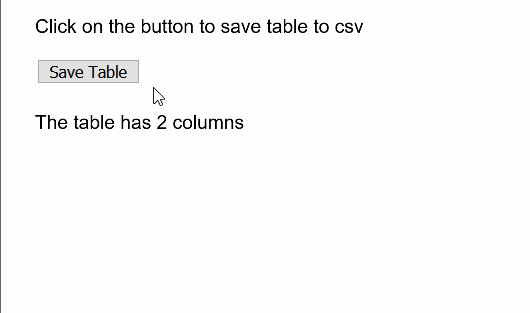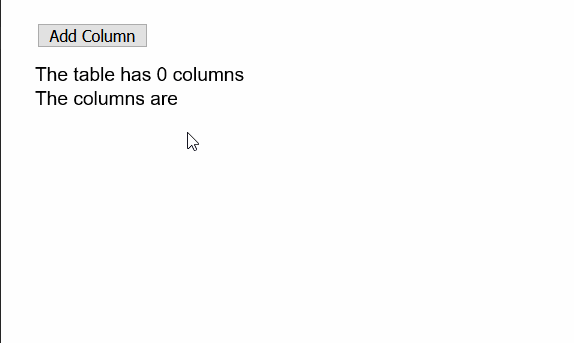# p5.js | p5.Table addColumn() Method

The addColumn() method of p5.Table in p5.js is used to add a new column to a table object. The method accepts a single parameter that is used to specify a title to the column so that it could be easily referenced later. It is an optional value and not specifying a title would leave the new column’s title as null.
Syntax:

```addColumn( [title] )
```

Parameters: This function accepts a single parameter as mentioned above and described below:

• title: It is a String which denotes the title of the new column. It is an optional parameter.

The examples below illustrates the addColumn() function in p5.js:
Example 1:

## javascript

 `function` `setup() { ` `  ``createCanvas(500, 300); ` `  ``textSize(16); ` ` `  `  ``saveTableBtn = createButton(``"Save Table"``); ` `  ``saveTableBtn.position(30, 50); ` `  ``saveTableBtn.mouseClicked(saveToFile); ` ` `  `  ``text(``"Click on the button to save table to csv"``, 20, 20); ` `   `  `  ``// Create the table ` `  ``table = ``new` `p5.Table(); ` ` `  `  ``// Add two columns ` `  ``// using addColumn() ` `  ``table.addColumn(``"author"``); ` `  ``table.addColumn(``"language"``); ` ` `  `  ``// Add two rows ` `  ``let newRow = table.addRow(); ` `  ``newRow.setString(``"author"``, ``"Dennis Ritchie"``); ` `  ``newRow.setString(``"language"``, ``"C"``); ` ` `  `  ``newRow = table.addRow(); ` `  ``newRow.setString(``"author"``, ``"Bjarne Stroustrup"``); ` `  ``newRow.setString(``"language"``, ``"C++"``); ` ` `  `  ``text(``"The table has "` `+ table.getColumnCount() +  ` `       ``" columns"``, 20, 100); ` `} ` ` `  `function` `saveToFile() { ` `  ``saveTable(table, ``"saved_table.csv"``); ` `} `

Output:Example 2:

## javascript

 `function` `setup() { ` `  ``createCanvas(600, 300); ` `  ``textSize(16); ` ` `  `  ``addColBnt = createButton(``"Add Column"``); ` `  ``addColBnt.position(30, 20); ` `  ``addColBnt.mouseClicked(addNewCol); ` ` `  `  ``// Create the table ` `  ``table = ``new` `p5.Table(); ` `} ` ` `  `function` `addNewCol() { ` `  ``let colName = ``"Random Column "` `+ floor(random(1, 100)); ` ` `  `  ``// Add the given column to table ` `  ``table.addColumn(colName); ` `} ` ` `  `function` `draw() { ` `  ``clear(); ` ` `  `  ``// Show the total number of columns ` `  ``// and current column names ` `  ``text(``"The table has "` `+ table.getColumnCount() +  ` `       ``" columns"``, 20, 60); ` `  ``text(``"The columns are"``, 20, 80); ` `  ``for` `(let i = 0; i < table.columns.length; i++) { ` `    ``text(table.columns[i], 20, 100 + i * 20); ` `  ``} ` `} `

Output:Online editor: https://editor.p5js.org/
Environment Setup: https://www.geeksforgeeks.org/p5-js-soundfile-object-installation-and-methods/My Personal Notes arrow_drop_upCheck out this Author's contributed articles.

If you like GeeksforGeeks and would like to contribute, you can also write an article using contribute.geeksforgeeks.org or mail your article to contribute@geeksforgeeks.org. See your article appearing on the GeeksforGeeks main page and help other Geeks.

Please Improve this article if you find anything incorrect by clicking on the "Improve Article" button below.

Article Tags :

Be the First to upvote.

Please write to us at contribute@geeksforgeeks.org to report any issue with the above content.